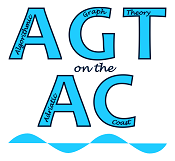#Algorithmic Graph Theory on the Adriatic Coast

Jun 16 – 19, 2015
UP FAMNIT, Koper, Slovenia
UTC timezone

## Scientific Programme with Timetable

Timetable: PDF

Algorithmic Graph Theory on the Adriatic Coast is an international workshop giving an opportunity to participants to present latest advances in Algorithmic Graph Theory and related areas.

The workshop will feature four invited talks:
• Endre Boros, Generation of monotone graph structures

• Vadim V. Lozin, From Matchings to Independent Sets

• Sang-il Oum, Constructive algorithm for rank-width of graphs and path-width/branch-width of matroids

• Dimitrios Thilikos, Algorithms and Combinatorics on the Erdős–Pósa property
There will also be a number of contributed talks.

Topics of interest include (but are not limited to):
• Graph decompositions
• Graph width parameters (treewidth, clique-width, rank-width, etc.)
• Graph modification problems
• Structural graph theory (structure of graphs in hereditary, monotone and minor-closed graph classes)
• Computational complexity of graph problems - classical and parameterized
• Approximation algorithms for graph problems
• Exact exponential-time algorithms for graph problems
• Graph classes related to perfect graphs
• Graph invariants - bounds, algorithmic aspects
• Planar graphs and generalizations
• Algorithmic aspects of symmetric graphs
• Algorithmic aspects of product graphs
• Algorithmic aspects of metric graph theory
• Applications of algorithmic graph theory